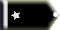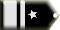# Standard temp on climb chartn9xv Trainee

I have an actual C-172 skyhawk POH. In the climb profile chart (TIME- FUEL-DISTANCE to climb) it uses a "standard" temperature of 0-deg. C to compute the correction factor for non-standard temperature conditions. Why are they not using 15-deg. C as the ISA standard?

Kevinn9xv TraineeCRJCapt Chief Captain

Can you post a picture of the chart? I have a 1977 Cessna 150 manual and the climb chart starts at sea level, 15 degrees Celsius. It's labeled Time, fuel and distance to climb. It ranges from S.L to 12,000 feet.n9xv Trainee

My chart is basically the same, but the verbage in their FUEL REQUIRED example is a bit muddy. It reads like this -

These values are for a standard temperature and are sufficiently accurate for most flight planning purposes. However, a further correction for the effect of temperature may be made as noted on the climb chart. The approximate effect of a non-standard temperature is to increase the TIME-FUEL-DISTANCE to climb by 10-% for each 10-deg C above standard temperature,due to the lower rate of climb. In this case, assuming a temperature 16-deg. C above standard (16 C - 0 C), the correction would be:

(16 C / 10 C) X 10-% = 16-% increase.

- When they say "a standard temperature", do they mean 15-deg. C as the ISA standard and a lapse rate of 2-deg. C per 1000-Ft.?

- When they say "16-deg. C above standard", do they mean 16-deg. above what the ISA standard lapse rate would have been? In other words, at 5000-Ft. for instance, the temperature per ISA standard lapse rate would be 49-deg. C. Do they mean 16-Deg. C above that? Which would be 65-deg. C.

- Where did the 0-deg. C in "(16-deg. C - 0-deg. C) come from?

There is no actual indication of 15-deg. C on the chart. They just start at sea level and go up to 12000-Ft.

I understand how to interpret the chart and the math involved, but I'm not clear on the terms.CRJCapt Chief Captain

n9xv wrote:

1. - When they say "a standard temperature", do they mean 15-deg. C as the ISA standard and a lapse rate of 2-deg. C per 1000-Ft.?

2. - When they say "16-deg. C above standard", do they mean 16-deg. above what the ISA standard lapse rate would have been? In other words, at 5000-Ft. for instance, the temperature per ISA standard lapse rate would be 49-deg. C. Do they mean 16-Deg. C above that? Which would be 65-deg. C.

3. - Where did the 0-deg. C in "(16-deg. C - 0-deg. C) come from?

4.-There is no actual indication of 15-deg. C on the chart. They just start at sea level and go up to 12000.

1. Yes, 15 Celsius(C) is standard temp at S.L and 5 C is standard temp at 5000 ft. MSL. Lets stick to on scale, Celsius (C).
2. They mean 16 degrees above the standard temperature for the altitude that the climb starts at.(S.L ISA +16 would equal 31C) You would increase your figures by 16%. 5000 ft. MSL would be 21 C with the same percent increase.
3. It's just an example. Climb is based on MSL not AGL heights. Field elevation could be 6500 ft. MSL. ISA temp. would be 0 C.
4. Standard temperature is 15 degrees C at S.L even if it's not marked.n9xv Trainee

Ok, so the standard is always understood to be the typical ISA standard. I thought thats what they must have meant but wanted to be sure. Thats a nice altitude chart too! Thats a keeper for the ole formula & data file. 🙂

While on a the subject of atmospheric stuff, do you know any good links on the Koch chart. I've always wondered if there were any formulas that could be implimented in a spread for such. Anyway, if you stumble onto something, pass it along. Thanks for the clarification on the climb chart too.

All times are GMT Page 1 of 1